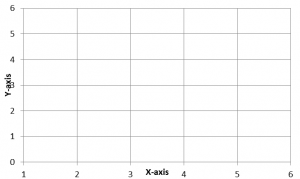Coordinates and Angles Worksheet and Explanation

We’ve structured this information to help children with their education. It is targetted at children in years 5 and 6 and the questions for the worksheet have been stripped from past papers. Having an Understanding of coordinates and angles is part of the primary school curriculum and children will deal with coordinates and angles in both KS1 and KS2.

The Information below will give an overview of the topic and we have included a detailed worksheet with full answers. The worksheet includes 31 coordinates and angles questions and is relevant for KS2 pupils approaching their SATS test.

If you are not ready to download the worksheets yet, then read on for some information about coordinates and angles. This has provided to introduce the topics covered in the worksheet for those that might be unfamiliar but also as a quick revision tool for those that would like a quick refresher before accessing the worksheet.

Coordinates Basics

Before explaining how we can plot co-ordinates it is important that we understand x-y graph plots as this will be the basis upon which we will be plotting these coordinates.Above we have an x-y plot. As you can see it has a horizontal x-axis along the bottom and a vertical y-axis up the side of the graph.

How to Plot Coordinates

Coordinates are expressed in the form (X,Y). The x coordinate is the number on the right-hand side of the brackets and they coordinate is the number on the left-hand side of the brackets. An easy way to remember this is ‘Along the corridor and up the stairs’. Meaning you plot the horizontal x-axis first followed by the vertical y-axis.

Practise Plotting Some Coordinates

On the graph above practice by plotting the points (2,2), (5,2), (5,4) and (2,5). What shape would be made if you joined all these points together? Answer: a rectangle!

Angles Basics

Angles are a measurement of the space between two lines that meet and are measured in degrees. The most common angles in you will see in KS1 and KS2 are right-angle or 90° which are the internal angles of squares and rectangles.

In KS1 children will start to learn about angles through learning about whole, half and quarter turns. this will then be developed to explain the direction they are going in: clockwise or anti-clockwise. In KS2 obtuse (angles greater than 90°) and acute angles (less than 90°) will be introduced and children will start using a protractor to measure angle size.